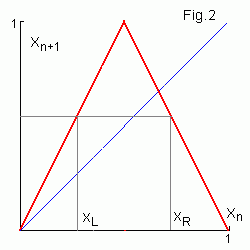# Tent map dynamicsConsider continuous tent map fc(x)
 f(x) = { cx , c(1 - x) , 0 ≤ x ≤ 1/2 1/2 < x ≤ 1
for c = 2. Similar to sawtooth map in the binary number system multiplying x by 2 corresponds to the left shift by one bit site. If before the shift the upper bit b1 = 1 (i.e. x ≥ 1/2) then (using 2 = 1.111...2 = 1.(1)2 ) we get
f(x) = 2 - 2x = 1.111... - 1.b2 b3 b4 ... = 0.u2 u3 u4 ... .
where uk = 1 - bk is inversion of the bit bk. Thus after the left shift the upper bit is truncated again but if it is 1 then all the rest bits are inverted. It is evident that symbolic sequence σ = (s1, s2, s3 ...) is obtained from the truncated bits.

## Conversion of symbolic sequences to coordinate X

To get rules for conversion of symbolic sequences to coordinate it is useful to recode bits of x by means of substitution 0 → 1 and 1 → -1. Then multiplication of bits by -1 inverts them. Therefore after the fist left shift it is enough to multiply all the bits by the first one p1 and so on. Then the tent map dynamics is
 0 . p1   p2   p3   p4 ... s1= p1 . (p1 p2 )   (p1 p3 )   (p1 p4 ) ... s2= p1 p2 . (p1 p2 p1 p3 )   (p1 p2 p1 p4 ) ...   or taking into account that pk2 = 1 . (p2 p3 )   (p2 p4 ) ... s3= p2 p3 . (p3 p4 )   (p3 p5 ) ... ... sn= pn-1 pn . (pn pn+1 )   (pn pn+2 ) ...
For the n-th term of the corresponding symbolic sequence we get
sn = pn-1 pn .
Multiplying this formula by pn-1 we get
pn = sn pn-1 ,   p1 = s1 .
By means of this formula we get x recursively from symbolic sequence. In the standard notation these rules are
 bn = { bn-1 1 - bn-1 if   sn = 0 if   sn = 1
For example symbolic sequence σ = {111...} = (1) corresponds to the unstable fixed point x1 = 0.(10) = 102 /112 = 2/3. For period-2 orbit σ = (01) we get x1 = 0.(0110) = 01102 /11112 = 2/5 and x2 = 0.(1100) = 11002 /11112 = 4/5.
Now you can repeat all the "chaos story" told for the sawtooth map before. Later it will be repeated for the quadratic map for c = -2.
Contents   Previous: Sawtooth map   Next: Strange Cantor repeller
updated 12 July 2006